/

### The Laplace distribution

The Laplace distribution is akin to two exponential distributions spliced together.

 Distribution name Laplace distribution Common notation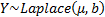Parameters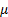= location parameter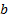= scale parameter (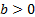) Domain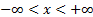Probability density function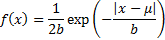Cumulative distribution function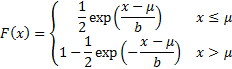MeanVariance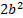Skewness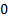(Excess) kurtosis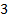Characteristic function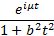Other comments The median and mode are. The inverse cdf (i.e. quantile) function is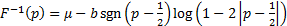.   If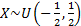and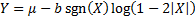then.   It is sometimes referred to as the double exponential distribution (but this term is also apparently also used sometimes of the Gumbel distribution)

Nematrian web functions

Functions relating to the above distribution may be accessed via the Nematrian web function library by using a DistributionName of “laplace”. For details of other supported probability distributions see here.